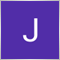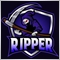# Order Modify error 4756 (10013 - invalid request)53

Hi

I am writing some code to try modifying my order , I keep getting this message in the strategy tester

failed modify order #2 buy 0.00  at market sl: 0.00000 tp: 0.00000 -> 1.28769 (1.28669), sl: 1.27452 tp: 1.29569 [Invalid request]

the trade is being opened normally but modification fails

can anyone help with this , attached below is the code i am trying

```#include <Trade\Trade.mqh>
//+------------------------------------------------------------------+
//| Expert initialization function                                   |
//+------------------------------------------------------------------+
int OnInit()
{
//---
//---
return(INIT_SUCCEEDED);
}

//+------------------------------------------------------------------+
//| Expert tick function                                             |
//+------------------------------------------------------------------+
void OnTick()
{
//---

double bidNow =NormalizeDouble(SymbolInfoDouble(_Symbol,SYMBOL_BID),_Digits);

double equity = AccountInfoDouble(ACCOUNT_EQUITY);

double balance = AccountInfoDouble(ACCOUNT_ASSETS);

double SL = bidNow-(500*_Point);

//  open orders
{

modifyOrder(price,SL,TP);

}
}
//+------------------------------------------------------------------+

void modifyOrder(double price, double  SL, double TP )
{

double stopLoss = SL-(500*_Point) ;
double takeProfit  = TP+(500*_Point);
double nPrice = price+(200*_Point);
double slimit =  price+(100*_Point);

{

Print( "modified" + GetLastError());

} else

{
Print("unable to modify " + GetLastError());
}

}```13

I am with the same problem, if you already sove this could you show it here? I'll do it if I find a way to fix it.

Thank you.41

I don't use cTrade, but it seems you buy something and then want to change the price of it? Buy is not a pending order, I guess?21

hello, do you have a solution for this issue? now i have the same problem.

thanks.468

i guess u need to trigger that from a custom button